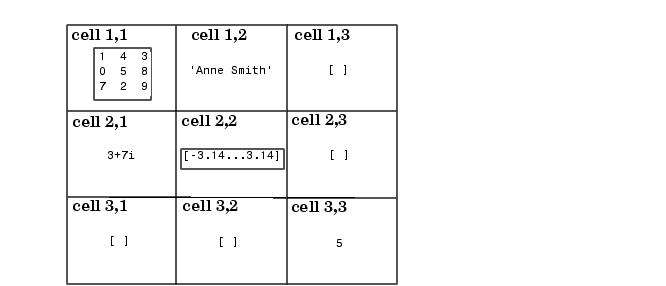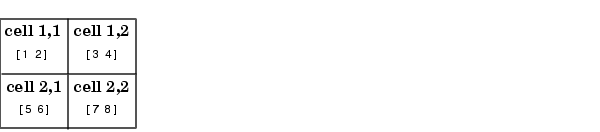ProgrammingCreating Cell Arrays

You can create cell arrays by

• Using assignment statements
• Preallocating the array using the `cell` function, then assigning data to cells

Creating Cell Arrays with Assignment Statements

You can build a cell array by assigning data to individual cells, one cell at a time. MATLAB automatically builds the array as you go along. There are two ways to assign data to cells:

• Cell indexing
• Enclose the cell subscripts in parentheses using standard array notation. Enclose the cell contents on the right side of the assignment statement in curly braces `{}`. For example, create a 2-by-2 cell array `A`:

• ```A(1,1) = {[1 4 3; 0 5 8; 7 2 9]};
A(1,2) = {'Anne Smith'};
A(2,1) = {3+7i};
A(2,2) = {-pi:pi/10:pi};
```

 Note    The notation `{}` denotes the empty cell array, just as `[]` denotes the empty matrix for numeric arrays. You can use the empty cell array in any cell array assignments.

• Content indexing
• Enclose the cell subscripts in curly braces using standard array notation. Specify the cell contents on the right side of the assignment statement:

• ```A{1,1} = [1 4 3; 0 5 8; 7 2 9];
A{1,2} = 'Anne Smith';
A{2,1} = 3+7i;
A{2,2} = -pi:pi/10:pi;
```

The various examples in this guide do not use one syntax throughout, but attempt to show representative usage of cell and content addressing. You can use the two forms interchangeably.

 Note    If you already have a numeric array of a given name, don't try to create a cell array of the same name by assignment without first clearing the numeric array. If you do not clear the numeric array, MATLAB assumes that you are trying to "mix" cell and numeric syntaxes, and generates an error. Similarly, MATLAB does not clear a cell array when you make a single assignment to it. If any of the examples in this section give unexpected results, clear the cell array from the workspace and try again.

MATLAB displays the cell array `A` in a condensed form:

• ```A =
[3x3 double]    'Anne Smith'
[3.0000+ 7.0000i]    [1x21 double]
```

To display the full cell contents, use the `celldisp` function. For a high-level graphical display of cell architecture, use `cellplot`.

If you assign data to a cell that is outside the dimensions of the current array, MATLAB automatically expands the array to include the subscripts you specify. It fills any intervening cells with empty matrices. For example, the assignment below turns the 2-by-2 cell array `A` into a 3-by-3 cell array.

• ```A(3,3) = {5};```

Cell Array Syntax: Using Braces

The curly braces `{}` are cell array constructors, just as square brackets are numeric array constructors. Curly braces behave similarly to square brackets, except that you can nest curly braces to denote nesting of cells (see Nesting Cell Arrays for details).

Curly braces use commas or spaces to indicate column breaks and semicolons to indicate row breaks between cells. For example,

• ```C = {[1 2], [3 4]; [5 6], [7 8]};
```

results inUse square brackets to concatenate cell arrays, just as you do for numeric arrays.

Preallocating Cell Arrays with the cell Function

The `cell` function allows you to preallocate empty cell arrays of the specified size. For example, this statement creates an empty 2-by-3 cell array:

• ```B = cell(2, 3);
```

Use assignment statements to fill the cells of `B:`

• ```B(1,3) = {1:3};
```

The `cell` function offers the most memory-efficient way of preallocating a cell array.

Memory Requirements for Cell Arrays

You do not necessarily need a contiguous block of memory to store a cell array. The memory for each cell needs to be contiguous, but not the entire array of cells.Cell Arrays Obtaining Data from Cell Arrays© 1994-2005 The MathWorks, Inc.